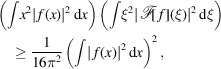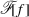International
Tables for
Crystallography
Volume B
Reciprocal space
Edited by U. Shmueli

International Tables for Crystallography (2010). Vol. B, ch. 1.3, p. 39   | 1 | 2 |

## Section 1.3.2.4.4.3. Heisenberg's inequality, Hardy's theorem

G. Bricognea

aGlobal Phasing Ltd, Sheraton House, Suites 14–16, Castle Park, Cambridge CB3 0AX, England, and LURE, Bâtiment 209D, Université Paris-Sud, 91405 Orsay, France

#### 1.3.2.4.4.3. Heisenberg's inequality, Hardy's theorem

| top | pdf |

The result just obtained, which also holds for, shows that the peakier', the broader'. This is a general property of the Fourier transformation, expressed in dimension 1 by the Heisenberg inequality (Weyl, 1931):where, by a beautiful theorem of Hardy (1933), equality can only be attained for f Gaussian. Hardy's theorem is even stronger: if both f andbehave at infinity as constant multiples of G, then each of them is everywhere a constant multiple of G; if both f andbehave at infinity as constant multiples of, then each of them is a finite linear combination of Hermite functions. Hardy's theorem is invoked in Section 1.3.4.4.5to derive the optimal procedure for spreading atoms on a sampling grid in order to obtain the most accurate structure factors.

The search for optimal compromises between the confinement of f to a compact domain in x-space and ofto a compact domain in ξ-space leads to consideration of prolate spheroidal wavefunctions (Pollack & Slepian, 1961; Landau & Pollack, 1961, 1962).

### References

Hardy, G. H. (1933). A theorem concerning Fourier transforms. J. London Math. Soc. 8, 227–231.
Landau, H. J. & Pollack, H. O. (1961). Prolate spheroidal wave functions, Fourier analysis and uncertainty (2). Bell Syst. Tech. J. 40, 65–84.
Landau, H. J. & Pollack, H. O. (1962). Prolate spheroidal wave functions, Fourier analysis and uncertainty (3): the dimension of the space of essentially time- and band-limited signals. Bell Syst. Tech. J. 41, 1295–1336.
Pollack, H. O. & Slepian, D. (1961). Prolate spheroidal wave functions, Fourier analysis and uncertainty (1). Bell Syst. Tech. J. 40, 43–64.
Weyl, H. (1931). The Theory of Groups and Quantum Mechanics. New York: Dutton. [Reprinted by Dover Publications, New York, 1950.]